Ex 13.2

Chapter 13 Class 11 Limits and Derivatives
Serial order wise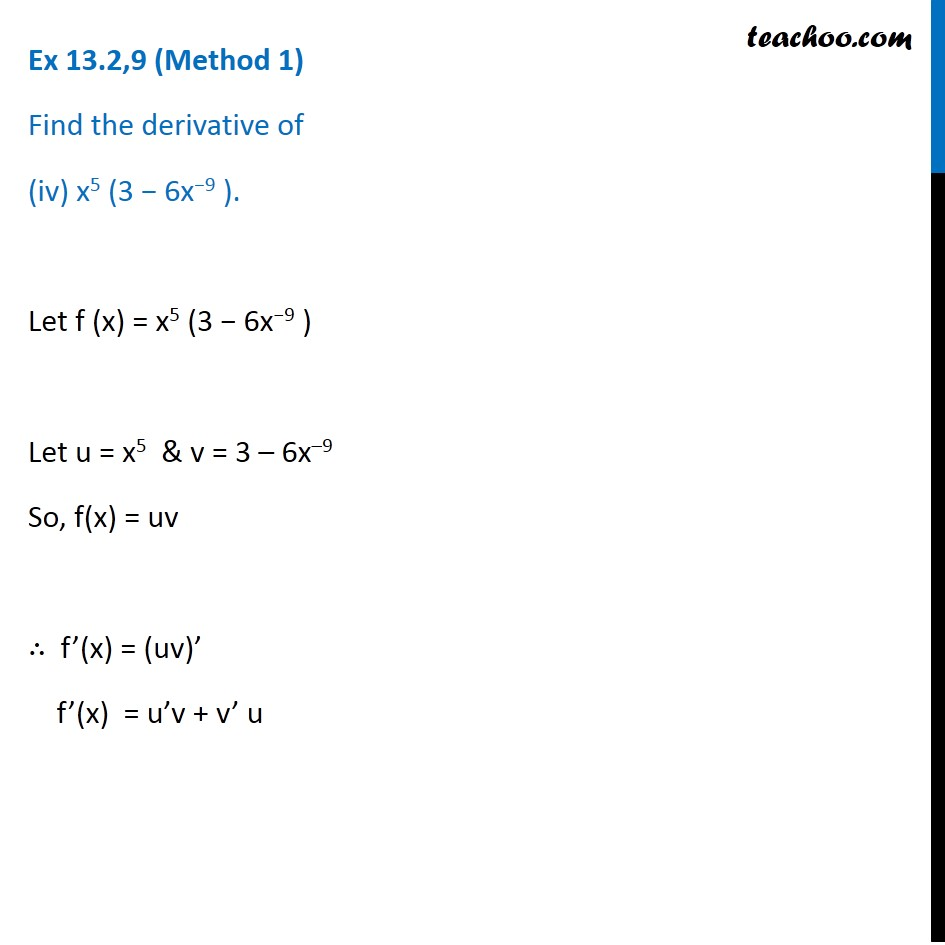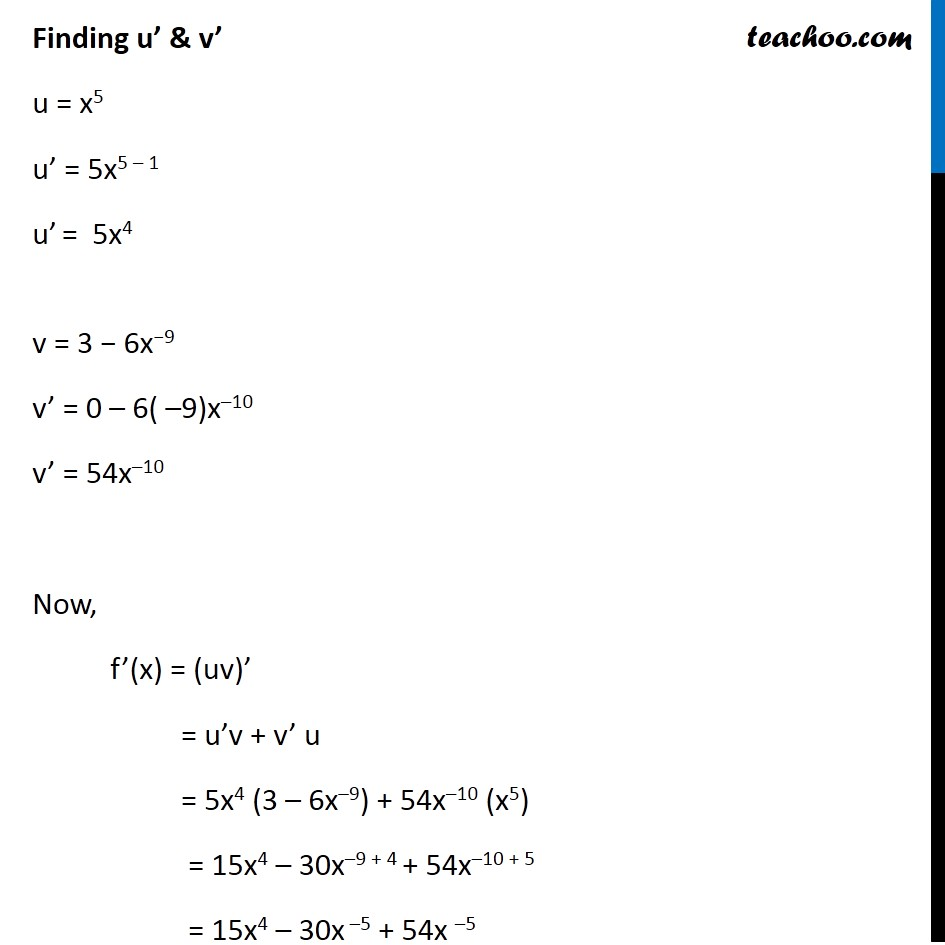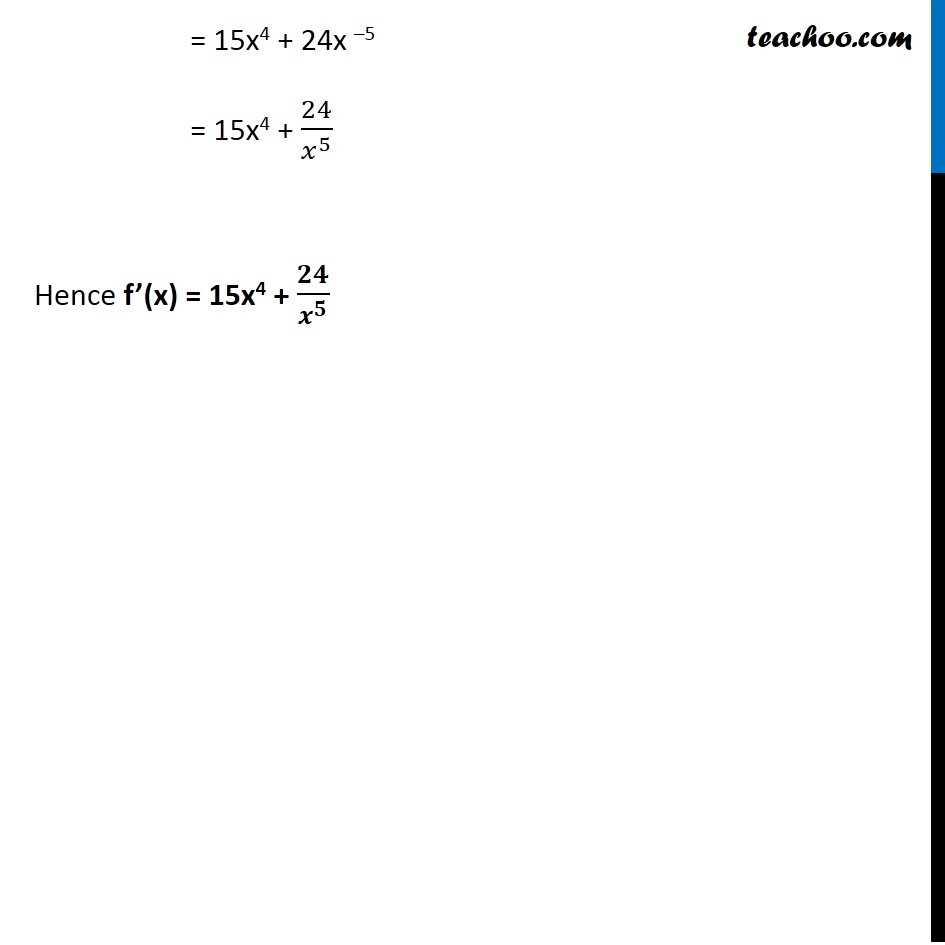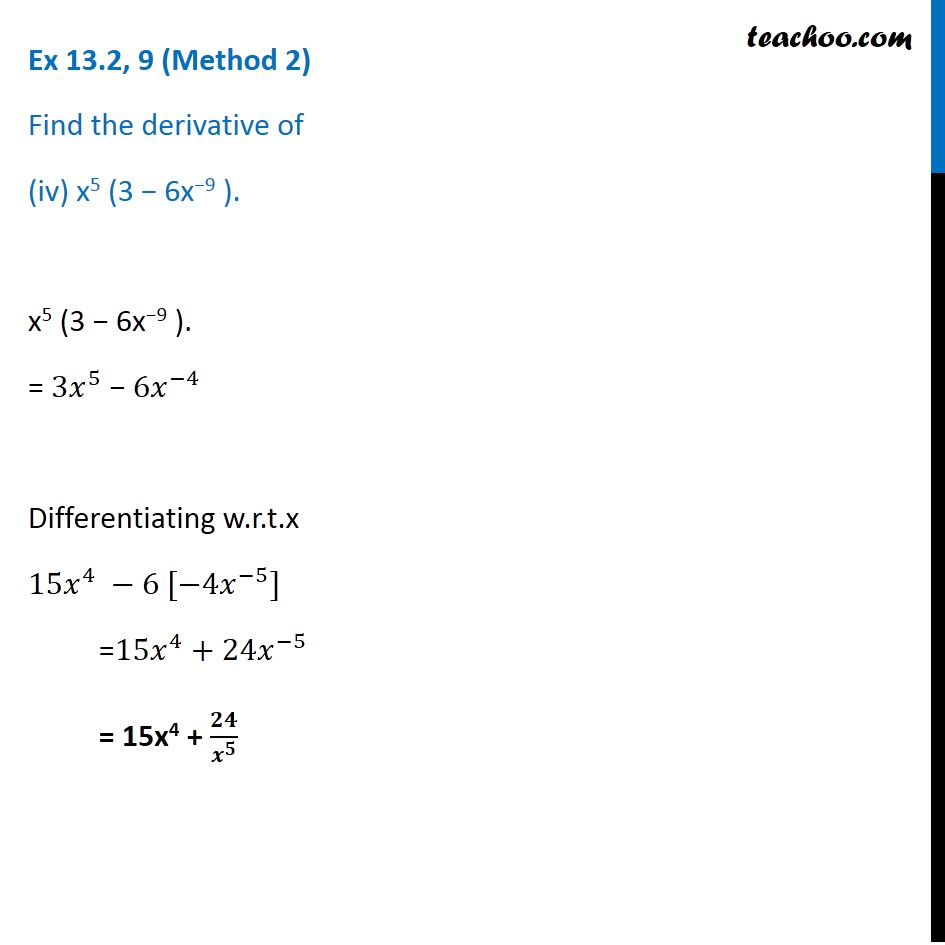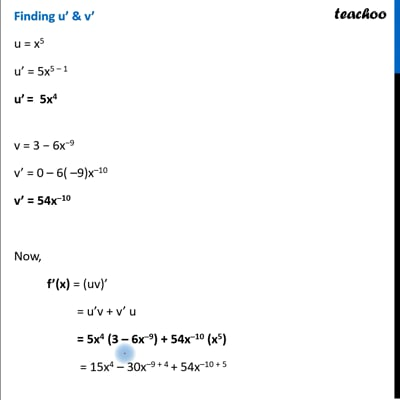This video is only available for Teachoo black users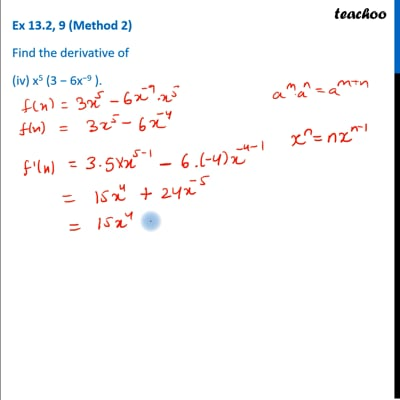This video is only available for Teachoo black users

Get live Maths 1-on-1 Classs - Class 6 to 12

### Transcript

Ex 13.2,9 (Method 1) Find the derivative of (iv) x5 (3 − 6x−9 ). Let f (x) = x5 (3 − 6x−9 ) Let u = x5 & v = 3 – 6x–9 So, f(x) = uv ∴ f’(x) = (uv)’ f’(x) = u’v + v’ u Finding u’ & v’ u = x5 u’ = 5x5 – 1 u’ = 5x4 v = 3 − 6x−9 v’ = 0 – 6( –9)x–10 v’ = 54x–10 Now, f’(x) = (uv)’ = u’v + v’ u = 5x4 (3 – 6x–9) + 54x–10 (x5) = 15x4 – 30x–9 + 4 + 54x–10 + 5 = 15x4 – 30x –5 + 54x –5 = 15x4 + 24x –5 = 15x4 + 24x –5 = 15x4 + 24/𝑥^5 Hence f’(x) = 15x4 + 𝟐𝟒/𝒙^𝟓 Ex 13.2, 9 (Method 2) Find the derivative of (iv) x5 (3 − 6x−9 ). x5 (3 − 6x−9 ). = 〖3𝑥〗^5 − 〖6𝑥〗^(−4) Differentiating w.r.t.x 〖15𝑥〗^4 −6 [−4𝑥^(−5)] =〖15𝑥〗^4+24𝑥^(−5) = 15x4 + 𝟐𝟒/𝒙^𝟓